What does it do?

Calculates the number of Days, Months, or Years between two dates

Formula breakdown:

=DATEDIF(Start Date,End Date,Interval)

What it means:

=DATEDIF(starting date, ending date, the unit of measurement)

Where INTERVAL is:

m” Months , “ym” Months Excluding Years
d” Days , “yd” Days Excluding Years
y” Years , “md” Days Excluding Years And Months

The DATEDIF function is a mystery function within Excel.  When you write it out in a workbook it doesn’t give you any hints like other functions would and if you look it up in the function list you would not find it! Creepy…

The DATEDIF function stands for “date difference” and it calculates the number of Days, Months, or Years between two dates.

So if you want to find out how many days, years or months have passed since you were born, well this is the formula for you!   Well you can also extend this to project start and end dates, but you get my point.STEP 1: Enter the Start Date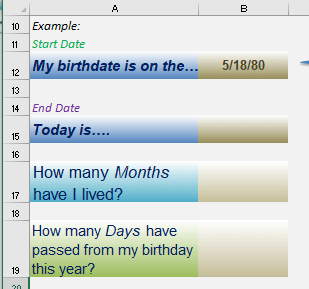STEP 2: Enter the End Date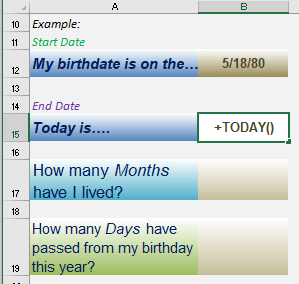STEP 3: To get the number of months as a difference, type in the following formula

## =DATEDIF(B12, B15, “m”)

The DATEDIF arguments:

B12 is the startdate
B15 is the enddate
m tells it to count in total months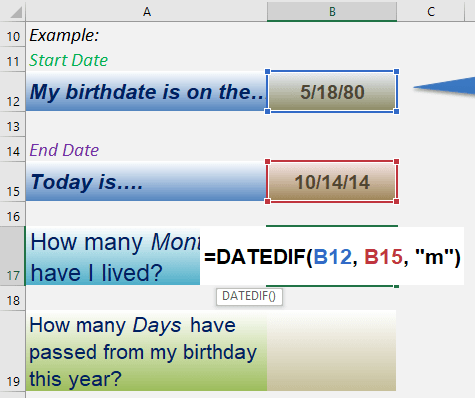STEP 4: To get the number of days on this year from your birthday, type in the following formula

## =DATEDIF(B12, B15, “yd”)

The DATEDIF arguments:

B12 is the startdate
B15 is the enddate
yd tells it to count in days but excluding the year portion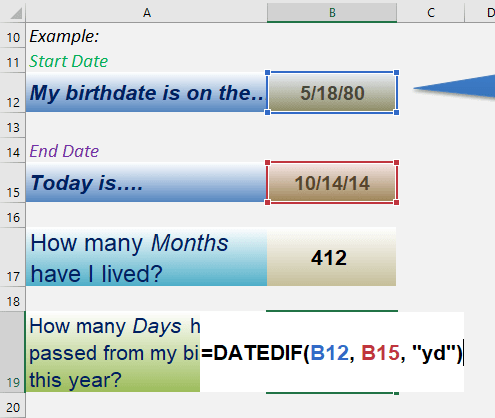And you have your calculated differences!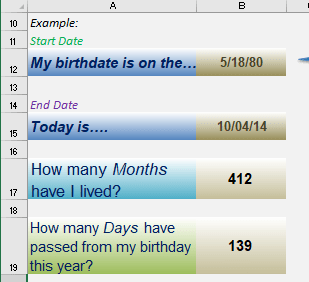How to Use the DateDif Formula in Excel